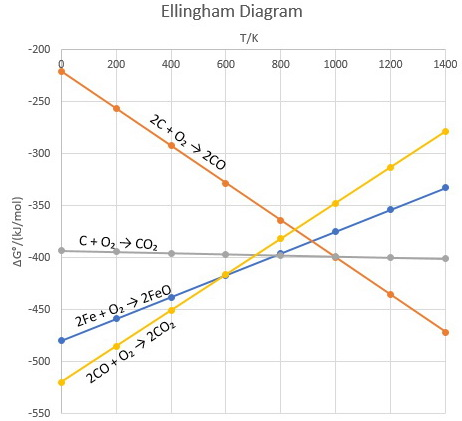# How to check ellingum diagram?

Gibbs equation helps us to predict the spontaneity of a reaction on the basis of enthalpy and entropy values directly. H.G.T Ellingham proposed the Ellingham diagram to predict the spontaneity of reduction of various metal oxides. Ellingham diagram was basically a curve which related the Gibbs energy value with the temperature. Gibbs energy is given as:

ΔG = ΔH – TΔS

Where ΔH is the change in enthalpy and ΔS is the change in entropy.

With respect to a reaction, Gibbs energy can be related to the equilibrium constant as:

ΔGo= – RTlnK

Where K is the equilibrium constant.

Thus, when the reaction is exothermic, enthalpy of the system is negative, thus making Gibbs free energy negative. Hence, we can say that the reaction will proceed in the forward direction due to a positive value of the equilibrium constant. This law can be scaled for two different reactions taking place in a system too.  The overall reaction (combination of two reactions) will occur if and only if net ΔG (sum of ΔG’s of both the reactions) of the two possible reactions is negative.

An Ellingham diagram is a plot of ΔG versus temperature for different reactions. For example,Thus, it is possible to predict the temperature above which, say, carbon or carbon monoxide will reduce any metal oxide, for example FeO.

Below 600 K, only CO reduces FeO.

Above 800 K, reduction by conversion of coke to carbon dioxide is spontaneous.

Above 900 K, reduction by conversion of coke to carbon monoxide is spontaneous.

Thus, for the reduction of FeO, carbon monoxide is the better reducing agent below 600 K, but carbon is the better reducing agent above 800 K.

### Preparation Products

##### Knockout JEE Main April 2021

An exhaustive E-learning program for the complete preparation of JEE Main..

₹ 22999/- ₹ 14999/-
##### Knockout JEE Main April 2022

An exhaustive E-learning program for the complete preparation of JEE Main..

₹ 34999/- ₹ 24999/-
##### Test Series JEE Main April 2021

Take chapter-wise, subject-wise and Complete syllabus mock tests and get in depth analysis of your test..

₹ 6999/- ₹ 4999/-
##### JEE Main Rank Booster 2021

This course will help student to be better prepared and study in the right direction for JEE Main..

₹ 13999/- ₹ 9999/-